Next: Physical Background Up: Introduction Previous: Mass Spectrum   Contents

# Line Width - Size Relation

Larson (1981) compiled the observations for molecular cloud complexes, molecular cloud and molecular clumps published in 1974-1979. He obtained an empirical relation that the size of a structure is well correlated to the random velocity in the structure which is measured by the width of the emission line (see Appendix E). Figure 1.28(left) shows this correlation and this is well expressed as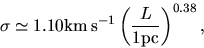(1.13)

where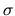and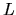represent respectively the three-dimensional random speed of gas and the size of the structure. A similar correlation is found only for giant molecular clouds (Sanders, Scoville, & Solomon 1985) as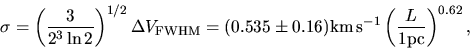(1.14)

for GMCs whose sizes are larger than 10pc (be careful the typos in their abstract: power was -0.62). He also found another correlation between the mass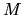and the random velocity like Figure 1.28(right), which is expressed as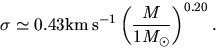(1.15)

In the next chapter (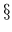2.9), we will see the virial relation, that is, for an isolated system to achieve a mechanical equlibrium the gravitaional to thermal energy ratio has to be equal to 2:1 for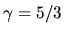gas. The ratio of the gravitational energy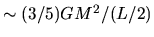to the thermal energy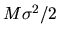is also fitted as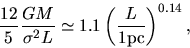(1.16)

which is weakly dependent of the size or the mass. This seems to mean the ratio is nearly constant irrespective of the mass or size of the clouds. Since there is a mutual relation between mass, size, and the velocity dispersion to achive a mechanical equlibrium (the Virial relation), there is only one independent correlation in the above two correlations (eqs.[1.13] and [1.15]). Although several reasons to explain the correlation are proposed, we have no consensus yet.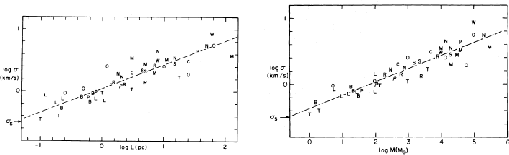Next: Physical Background Up: Introduction Previous: Mass Spectrum   Contents
Kohji Tomisaka 2007-07-08